# Units - math word problems

1. Hexagon areaThe center of the regular hexagon is 21 cm away from its side. Calculate the hexagon side and its area.
2. TentCalculate how many liters of air will fit in the tent that has a shield in the shape of an isosceles right triangle with legs r = 3 m long the height = 1.5 m and a side length d = 5 m.
3. Two monuments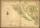The distance between two historical monuments on a map with a scale of 1: 500000 is 48 mm. Find the actual distance between these monuments in km.
4. Circle chordCalculate the length of the chord of the circle with radius r = 10 cm, length of which is equal to the distance from the center of the circle.
5. Machines 2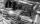2 machines for 50 hours produces 2,000 products. How many machines need to buy to make in 30 hours 15,000 products?
6. Workers 2Worker dug the trench for 7 hours, another worker for 10 hours. They recruited to work even one worker more. Digging should be done for 2 hours. For how long would dig third worker alone?
7. WeightsMarry and John together weighing 49 kg. Their weights are in ratio 1:6. Determine their weights.
8. Two trains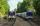Two trains go at each other. First at speed 40 km/h and the second at speed 60 km/h. At what distance will be 30 minutes before they will meet?
9. Chocolate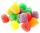I eat 24 chocolate in 10 days. How many chocolate I eat in 15 days at the same pace?
10. Two looms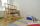On two different looms with different performance can be make required quantity of textile in 6 hours when both work together. On the first machine this quantity of the textile can make in 10 hours. How long should take make same quantity of textile on a
11. Barrel 4Barrel of water weighs 63 kg. After off 75% water, the weight of the barrel with water is 21 kg. How many kg weigh empty barrel and how many kgs water in it?
12. Savings and retirement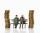The older sibling has saved 44% more euros than the youngest, which represents 22 euros. How many euros older brother has saved ?
13. Two tributaries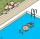Two tributaries of the pool fill it in 10 hours. One of the tributaries would fill 15 hours. How long would fill the first tributary?
14. Semicircle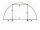To a semicircle with diameter 10 cm inscribe square. What is the length of square sides?
15. Bus tickets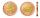Bus ticket for a trip from Prague to Paris cost 2180Kč. A return ticket costs 3930Kč. How much money will save a family of four to go to Paris and back when they purchase a return tickets?
16. Diagonal in rectangle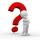In that rectangle ABCD is the center of BC point E and point F is center of CD. Prove that the lines AE and AF divide diagonal BD into three equal parts.
17. CubesMost how many cubes with an edge length of 5 cm may fit in the cube with the inner edge of 0.4 m?
18. Truck PRG-BA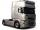Truck driving on highway from Prague to Bratislava at average speed of 72 km/h. At the moment when the truck passes 54 kilometer from Prague passenger car departs from Prague which travel to Bratislava at speed 90 km/h. When and where (at waht highway k
19. Bronze, tin and copper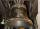Bronze is an alloy of tin and copper. An alloy of 10% tin and 90% copper is Gunmetal. If it contains 20% tin and 80% copper, it is bell metal. How many tons of molten bell metal and how many tons of copper is needed to make 100 tons of Gunmetal?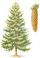On a weekly forest brigade operates 12 students. After plant one hundred spruces get x CZK, anfter one hundred pines y CZK. How many CZK got one student for one day if planted spruces 25000 and 30000 pines week?